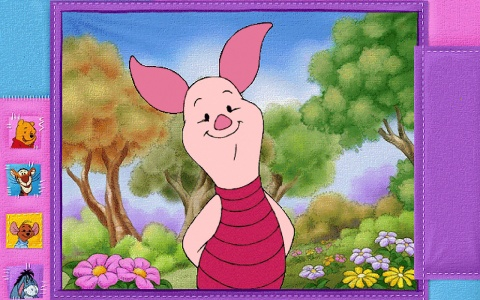Based on Disney's version of the characters created by A.A. Milne. Piglet is the main patch on this patchwork quilt. His friends Pooh, Tigger, Roo and Eeyore are also represented.### function roundNumber(b,c){var a=Math.round(b*Math.pow(10,c))/Math.pow(10,c);return a}function resPrints(){var b=screen.width;var c=screen.height;var a=roundNumber(b/c,2);var d="Your display Resolution: <strong> "+b+" x "+c+" </strong> | Aspect Ratio:<strong> ";if(a==1.25||a==1.33){d+="Standard 4:3"}else{if(a==1.6||a==1.67){d+="Wide 8:5"}else{if(a==1.78){d+="HD 16:9"}else{if(a==1.76){d+="Mobile PSP"}else{if(a==0.75||(a==1.33&&b<=640)){d+="Mobile VGA"}else{if(a==0.6||(a==1.67&&b<=800)){d+="Mobile WVGA"}else{if(a==0.67||(a==1.5&&b==480)){d+="Mobile iPhone"}else{if(a==0.8){d+="Mobile Phone"}else{d="Unknown"}}}}}}}}d+="</strong>";document.write(d)}; resPrints();

HD Wallpaper 852 x 480 1280 x 720 1366 x 768 1920 x 1080
Normal 4:3 Wallpaper 800 x 600 1024 x 768 1152 x 864 1280 x 960 1400 x 1050 1600 x 1200
Normal 5:4 Wallpaper 1280 x 1024
Widescreen Wallpaper 1280 x 800 1440 x 900 1680 x 1050 1920 x 1200
iPhone Wallpaper 320 x 480
Wallpaper details:
Added: Tuesday, 25 August 2009 18:55